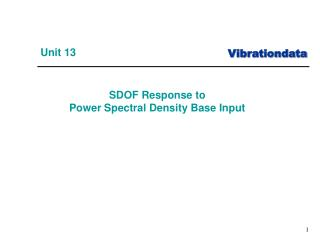Download PresentationUnit 13Unit 13 - PowerPoint PPT Presentation

Download PresentationUnit 13
An Image/Link below is provided (as is) to download presentation

Download Policy: Content on the Website is provided to you AS IS for your information and personal use and may not be sold / licensed / shared on other websites without getting consent from its author. While downloading, if for some reason you are not able to download a presentation, the publisher may have deleted the file from their server.

- - - - - - - - - - - - - - - - - - - - - - - - - - - E N D - - - - - - - - - - - - - - - - - - - - - - - - - - -
Presentation Transcript

1. Unit 13 • SDOF Response to Power Spectral Density Base Input

2. Exercise 5 Generate a white noise time history: Duration = 60 sec Std Dev = 1 Sample Rate=10000 Hz Lowpass Filter at 2500 Hz Save Signal to Matlab Workspace: white_60_input_th

3. Base Input Time History: white_60_input_th

4. Exercise 5 (cont) Generate the PSD of the 60-second white noise time history Input: white_60_input_th Use case 9 which has f  5 Hz Mean Removal Yes & Hanning Window Plot from 10 to 2000 Hz Save PSD to Matlab Workspace – white_60_input_psd

5. Base Input PSD: white_60_input_th The plateau is 0.0004 G2/Hz.

6. Recall SDOF Subjected to Base Input

7. SDOF Response to White Noise Subjected a SDOF System (fn=400 Hz, Q=10) to the 60-second white noise time history. Input: white_60_input_th Use Vibrationdata GUI option: SDOF Response to Base Input Save Acceleration Response time history to Matlab Workspace – pick a name

8. Response Time History: white_60_response_th

9. SDOF Response to White Noise PSD Take a PSD of the Response Time History Input: white_60_response_th Mean Removal Yes & Hanning Window Use case 8 which has f  5 Hz Plot from 10 to 2000 Hz Save Response PSD to Matlab Workspace: white_60_response_psd

10. Response PSD: white_60_response_psd

11. Plot Both PSDs Go to: Miscellaneous Functions > Plot Utilities Select Input > Two Curves Curve 1: white_60_input_psd Color: Red Legend: Input Curve 2: white_60_response_psd Color: Blue Legend: Response Format: log-log X-axis: 10 to 2000 Hz X-label: Frequency (Hz) Y-label: Accel (G^2/Hz)

12. The SDOF system response has unity gain at low frequencies, below, say 50 Hz. Dynamic amplification occurs at the 400 Hz natural frequency. Attenuation occurs at frequencies beginning near 600 Hz.

13. Matlab array name Power Transmissibility: trans Calculate Power Transmissibility from the response and input PSDs using the Vibrationdata GUI package. The peak has a magnitude of Q2=100, but this relationship usually only works for SDOF response. The 3 dB bandwidth method is more reliable for estimating the Q value.

14. Half-power Bandwidth Points (-3 dB) • f = (419.5-377.4) Hz • = 42.1 Hz • Viscous Damping Ratio • = f / (2 f ) • = 42.1/ (2*400) • = 0.0526 • Q = 1 / ( 2 * 0.0526 ) • Q = 9.5 • 5% lower than true value Q=10 Response PSD: white_60_response_psd

15. Curve-fit method using the Power Transmissibility Function Input Matlab array name: trans Miscellaneous Functions > Damping Functions > Half Power Bandwidth Damping This curve-fitting method is actually an extension of the half power bandwidth method.

16. Miles Equation The Miles equation is a simplified method of calculating the response of a single-degree-of-freedom system to a random vibration base input, where the input is in the form of a power spectral density. Furthermore, the Miles equation is an approximate formula which assumes a flat power spectral density from zero to infinity Hz. As a rule-of-thumb, it may be used if the power spectral density is flat over at least two octaves centered at the natural frequency.

17. Miles Equation The Miles equation is a simplified method of calculating the response of a single-degree-of-freedom system to a random vibration base input, where the input is in the form of a power spectral density. Furthermore, the Miles equation is an approximate formula which assumes a flat power spectral density from zero to infinity Hz. As a rule-of-thumb, it may be used if the power spectral density is flat over at least two octaves centered at the natural frequency.

18. Miles Equation (cont) The overall response acceleration is where fn = natural frequency P = PSD level at fn Q = amplification factor

19. Miles Equation Example SDOF System (fn = 400 Hz, Q=10) = 1.59 GRMS

20. Miles Equation, Relative Displacement The 3 relative displacement is inch where fn = natural frequency P = PSD G^2/Hz level at fn Q = amplification factor

21. Better Method We will learn a method that is better than Miles equation in an upcoming Webinar!